﻿ Boffin Access Limited - Open Access Journals - Scholarly Publishing-STEM# ADVANCES IN COMPUTER SCIENCES (ISSN:2517-5718)

### Tsang-Ling Sheu1 *, Kang-Wei Chang1, Fu-Ming Yeh2

1 Department of Electrical Engineering, National Sun Yat-Sen University, Kaohsiung, Taiwan
2The Broadband Wireless Department, Gemtek Technology Company, Hsinchu, Taiwan

### CitationCitation COPIED

Sheu TL, Chang KW, Yeh FM. A Dynamic Allocation Scheme for Resource Blocks using ARQ Status Reports in LTE Networks. Adv Comput Sci.2018 Jan: 1(1):102.

### Abstract

This paper presents a Dynamic RB (Resource Blocks) Allocation (DRBA) in LTE (LongTerm Evolution) networks by utilizing Automatic Repeated Request (ARQ). From the ARQ status report, an Evolved-Node Base Station (eNodeB) computes the successfully received packets per unit time for each User Equipment (UE). Thus, an eNB can adequately allocate Resource Blocks (RB) for a UE. In DRBA, we consider three different traffic types (audio, video, and data) with the priority from the highest to the lowest. To prevent the starvation of data traffic, we set an upper bound of RBs for audio and video traffic. To demonstrate the superiority of DRBA, we perform NS-3 simulation. Simulation results show that DRBA can perform much better than the traditional Automatic Modulation and Coding (AMC) scheme. In particular, when a UE is in high interference/noise environment, DRBA can achieve higher utilization, lower blocking rate, and admit more successfully connected UE.

### Keywords

RB; ARQ; LTE; Traffic Types; AMC

### Introduction

AMC (Automatic Modulation and Coding) scheme used in LTE (Long Term Evolution) networks can select an adequate modulation technique, such as BPSK, QPSK, and QAM, based on the channel quality of a UE (User Equipment) . From the requested data rate and the selected modulation technique, the number of RB (Resource Blocks) required for a UE can be determined . However, automatic modulation and coding (AMC), when allocating RB, does not take into account (i) the processing capability of a UE, and (ii) the unpredictable channel quality. Thus, in this paper, by estimating the processing capability of a UE and by adapting to the changeable channel quality, we propose a dynamic allocation scheme for RB by utilizing the status report of automatic repeated request (ARQ) .

Previous work on RB allocations in LTE networks can be divided into three categories. The first category allocates RB based on the requested Data Rate (DR) of UE. For examples, Maria, et al. proposed a fairness-based resource allocation in LTE downlink . Their scheme can achieve fairness while maintaining a higher throughput. Yet, the authors assumed the same upper bound for all channels, which is not realistic. Zhang, et al. proposed RB allocations with priority based on video encoding bit rate and the queuing length of UE . By considering a UE may be located in the overlapping area of two eNodeB, Wang, et al. proposed a method which allows two eNodeB to exchange their transmitting power and then decide which one is responsible for serving the UE . The second category allocates RB based on traffic types, such as real-time and non-real-time. Lee, et al. built a mathematical model to compute the system throughput by assuming that real-time traffic has the highest priority for RB allocations . Huo, et al. proposed RB allocations for both real-time and non-real-time traffic by using a utility function for achieving the fairness of subcarriers . The third category allocates RB based on AMC and channel quality indicator (CQI). For examples, Hou, et al. derives a new SINR equation by considering channel gains, total system RB, and signal intensity . Each UE is then allocated RB with different weights. Tham, et al . proposed a RB allocation scheme by considering CQI, traffic types, and the bit error rate (BER) reported by each UE . El-Hajj, et al. proposed a queue-aware mechanism, which can allocate RB based on the queue length of each UE . The authors proved their scheme can achieve better throughput as it is compared to a proportional fairness scheme. Aboul Hassan et al. and Ghosh et al. proposed RB allocations in multicell environment [12, 13]. Prior to RB allocations, neighboring eNBs will communicate about how to divide the shared bandwidth such that the interference can be kept in a minimum. Liu, et al. proposed a scheme to determine whether or not to allocate RB for the retransmission of erroneous packets . In other words, if packet retransmission delay exceeds its real-time constraint, RB for packet retransmission is not allocated. Finally, Zhu, et al. derives an objective function which can allocate different numbers of RB to different layers (such as base layer and enhancement layer) of a scalable video stream .

Unlike the previous work, in this paper, we propose a dynamic RB allocation (DRBA) in LTE networks. The major innovation of the proposed DRBA is right in that it fully utilizes the periodical ARQ status reports returned from a UE. Specifically, from the ARQ status report, the successfully received packets per unit time for each UE can be computed. Thus, an adequate amount of RB, which is equivalent to the effective data rate, can be allocated to each UE, by considering changeable channel qualities associated with different modulation techniques.

The remainder of this paper is organized as follows. In Section 2, we introduce RB allocations in an OFDMA frame of LTE. In Section 3, we design the dynamic RB allocations and derive the effective data rate according to the returned ARQ statue report from a UE. In Section 4, the simulation of DRBA on NS-3 is performed and the simulation results are discussed. Finally, conclusion remarks are drawn in Section 5.

### Resource Blocks In LTE

According to the standard of 3GPP, an OFDMA (Orthogonal Frequency Division Multiplexing Access) frame in LTE appears periodically every 10 msec . In an OFDMA frame, there are 20 times slots divided into 10 sub-frames. Thus, each sub-frame contains two time slots with one slot lasting for 0.5 msec. As shown in (Figure 1), a Resource Block (RB) comprises two dimensions, a time slot with 7 symbols and 12 sub-carriers. Notice that a Resource Element (RE), consisting of exactly one subcarrier and one symbol, is the smallest entity and the building block of RB (one RB contains 84 RE).

In this paper, to be more flexible in allocating RB for a UE, we assume the size of a RB may contain any number of RE, which can be computed from the requested data rate. Prior to the introduction of DRBA, we begin to review the conventional RB allocations based on AMC.

As shown in (Figure 2), depending on the Channel Quality Indicator (CQI), a modulation technique, such as BPSK, QPSK, QAM, etc, and the corresponding subcarriers are selected to meet the requested data rate of a UE. The conventional RB allocation uses the requested data rate and channel quality reported from a UE to compute the size of RB (in terms of the number of RE). In this paper, we refer to this conventional method as RB Allocated by AMC (RAMC). However, RAMC is not an effective RB allocation method, since channel quality may vary with time and change along with the movement of a UE. For a long-lived application, such as video streaming, the actual size of RB required by a UE may become smaller when channel quality becomes poorer. Thus, RAMC may just waste too much bandwidth due to excessive RB allocations, which harmfully confines the number of UE admitting to the LTE network. To compare with the size of RB allocated by RAMC, (Figure 2) illustrates the actual RB size in red color.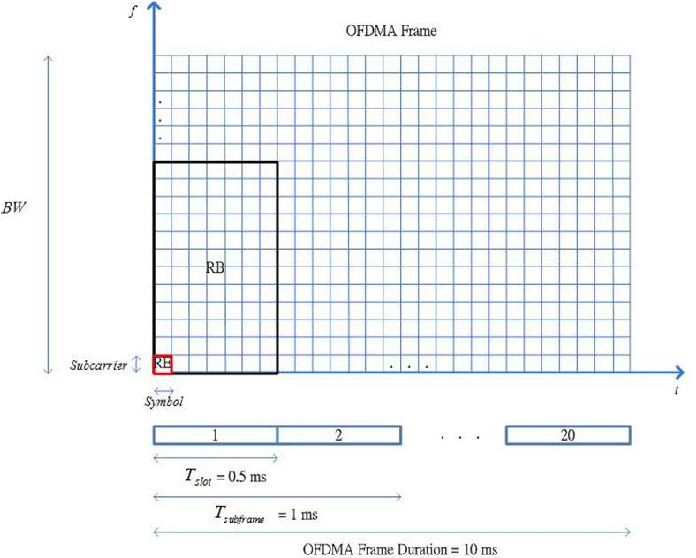Figure 1: RB in an OFDMA Frame of LTEIn this paper, to be more flexible in allocating RB for a UE, we assume the size of a RB may contain any number of RE, which can be computed from the requested data rate. Prior to the introduction of DRBA, we begin to review the conventional RB allocations based on AMC.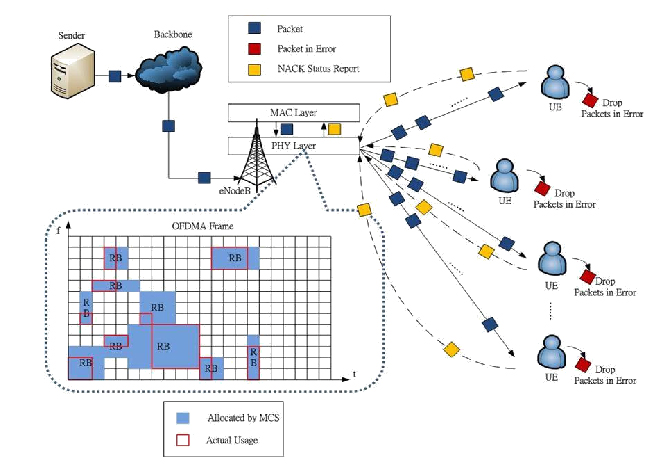Figure 2: RB allocations by AMC

### Dynamic RB Allocations

To remedy the excessive-allocation problem, in this paper we propose DRBA, which can dynamically compute the actual size of RB by utilizing the ARQ status report sent to eNodeB regularly from a UE.

Re-design of ARQ Status Report

ARQ status report in LTE networks is originally designed for eNodeB to retransmit the packets in error. Basically, it contains a negative ACK field, NACK_SN (10 bits), representing the sequence number of every erroneous packet. The original design of ARQ status report is not efficient for eNodeB to compute the number of successfully received packets by a UE.

To allow eNodeB more efficiently compute the number of packets successfully received by a UE, as shown in (Figure 3), we re-design the ARQ status report. Two new fields are added to the status report.

Size of RB vs Requested Data Rate
Once eNodeB knows the number of successfully received packets by a UE from the ARQ status report, the next step for eNodeB is to compute the size of RB (in terms of the number of RE) to be allocated to a UE. (Table 1) shows the parameters used in deriving an equation of the number of RE contained in a RB. First, let us compute the effective data rate ( Reff ) as in Eq. (3.1), where NRL represents the number of PDU (Protocol Data Unit) sent from eNodeB in ∆t , and NNACK represents the number PDU rejected by a UE in ∆t . Notice that both NRL and NNACK can be easily computed from the re-designed ARQ status report regularly transmitted from a UE.
Eq. (3.2) shows the symbol rate (Rsym), computed from the number of symbols (Nsym) in a slot and a slot duration (tslot). Eq. (3.3) shows the data rate of a RE (RRE), where l ave represents the average number of data bits carried by a symbol.
${\mathrm{{R}_{sym}=\frac{{N}_{sym}}{{t}_{slot}}}}_{}$                             (3.2)
(3.3)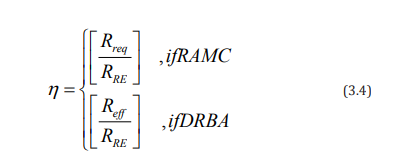Finally, we can derive the size of RB in terms of the number of RE (n) for RAMC and for DRBA, respectively, as shown in Eq. (3.4), where Rreq represents the initial requested data rate of a UE. As an example (Figure 4) illustrates the RB allocations using DRBA. We assume there are BW /f carriers, divided into l categories based on the CQI. Notice that l (1 ≤ l ≤ L) represents the number of bits carried by a symbol in OFDMA frames. RB in blue colors denotes that they are allocated by RAMC, and RB in purple colors denotes that they are allocated by DRBA. From Eq. (3.4), since Reff is smaller than Rreq, the size of RB in the latter is relatively smaller than the former.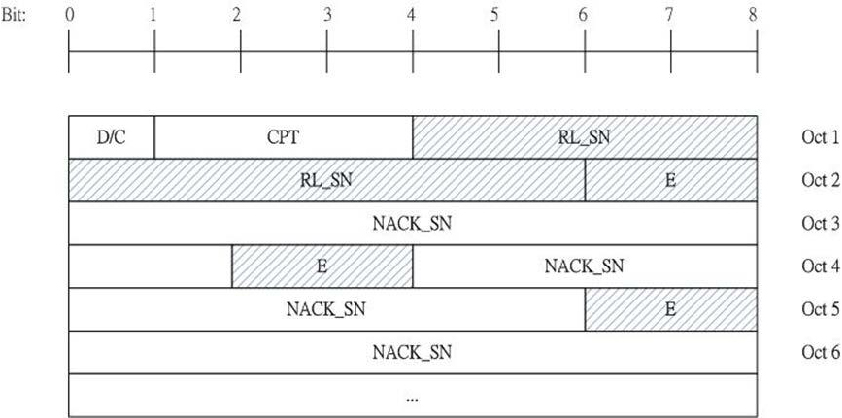Figure 3: Re-design of ARQ status report

They are RL_SN (10 bits), representing the largest sequence number received by a UE so far, and E (2 bits), representing that whether consecutive packets in error between two NACK_SN exist. Thus, with the help of RL_SN and two adjacent NACK_SN, the number of packets successfully received by a UE can be computed by eNodeB.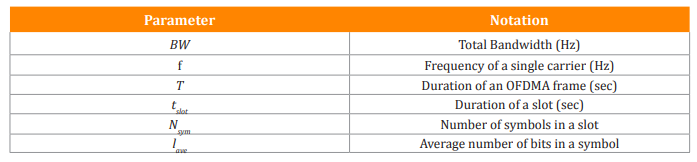Table 1: Parameters used in DRBA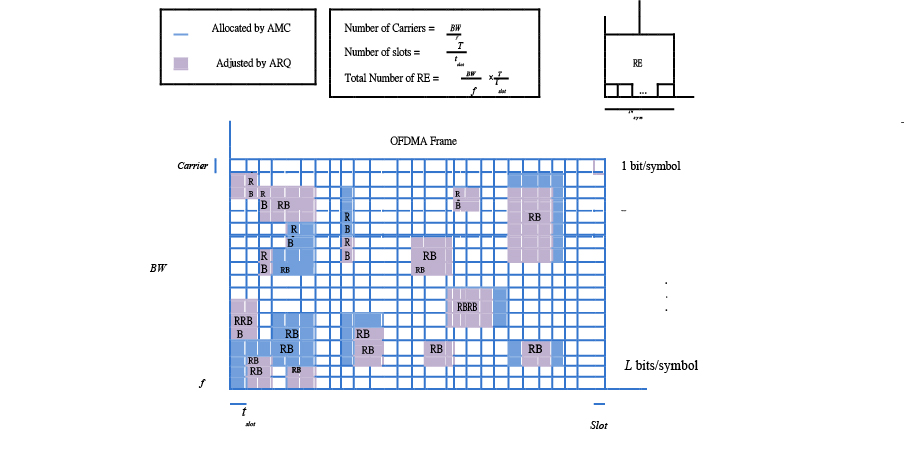Figure 4: RB allocations using DRBA

### Simulation and Discussions

To analyze the proposed DRBA, we perform simulation on NS-3 by embedding the LTE modules. (Table 2) shows the parameters and settings used in NS-3 simulation. Notice that we generate three types of multimedia traffic, audio, video, and data. Each type of traffic has different bit rates and durations.

(Figure 5) shows the comparison of average blocking rates between RAMC (allocated by AMC) and DRBA (adjusted by ARQ). A connection in an LTE network can be blocked due to the requested data rate (or the size of RB) cannot be granted. The average blocking rate is therefore defined as the average rate of unsuccessful connection of the three multimedia traffic, audio, video, and data. As the number of UE exceeds 20, we can observe that DRBA can reduce the average blocking rate by at least 20%. In particular, as the interference/noise (Figure 6) shows the comparison of utilization of OFDMA frames. As the number of UE is below 20, DRBA (adjusted by ARQ) has lower utilization than RAMC (allocated by AMC), no matter when the interference/noise is 5 dB or 15 dB. However, as the number of UE exceeds 25, the utilization of DRBA is much higher than that of RAMC. Notice that the difference in frame utilization between the two methods can increase from 10% to 20%. In summary, the higher of interference/noise, the higher of the difference.

As shown in (Figure 7), we compare the Successfully Connected UE (SCU) between the two methods, RAMC and DRBA, by changing the interference/noise from 5 dB to 15 dB. It is observed that as the sequence number of OFDMA frame increases from 200 to 1000, SCU largely increases from 15 to 25. Notice that as the interference/noise increases from 5 dB to 15 dB, SCU in DRBA can still reach near 25, but SCU in RAMC drops below 20. This is because in DRBA we can accurately compute the effective data rate of a UE, which save the  bandwidth for admitting more UE to the LTE network.

By varying the buffer size from 3 to 25 packets in a UE, in (Figure 8), we compare the SCU between the two methods. (Figure 8(a)) Shows the SCU as the interference/noise is fixed to 5 dB and (Figure 8(b)) shows the SCU as the interference/noise is fixed to 15 dB. In general, no matter when the buffer size is 3 or 25 in a UE, the former can reach higher SCU than the latter due to the increasing interference/noise. It is interesting to notice that as the interference/noise is small (5 dB), smaller buffer size (3 packets) can achieve higher SCU than larger buffer size (25 packets). As shown in (Figure 8(a)), this phenomenon is more significant in DRBA (adjusted by ARQ), as it is compared to RAMC (allocated by AMC). The reason for this ambiguity is quite straightforward; smaller buffer size in a UE will create larger packet loss ratio, which in DRBA implies the effective data rate should be re-adjusted to a smaller number. Consequently, a certain percentage of occupied bandwidth can be released and it is re-assigned to the newly connected UE. On the other hand, as shown in (Figure 8(b)), when the interference/noise increases to 15 dB, the two SCU for different buffer sizes do not exhibit big difference.

(Figure 9(a)) and (Figure 9(b)) show the comparison of RB allocations for an OFDMA frame using RAMC and DRBA, respectively. Here we consider 40 UEs intending to connect to the LTE network. The interference/noise is fixed to 5 dB. As it can be observed, RB allocations using RAMC has left more blank spaces than that using DRBA, which proves that the former exhibits lower utilization of an OFDMA frame. Additionally, the latter has fewer and smaller empty holes than the former, which demonstrates that the latter may allow more UE to be connected to the LTE network.